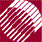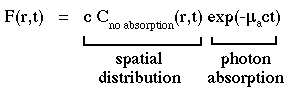ECE532 Biomedical Optics © 1998 Steven L. Jacques, Scott A. Prahl Oregon Graduate Institute

# Time-resolved Monte Carlo

## Separation of variables of spatial distribution and photon absorption

The above is calculated as if no absorption is present, i.e., µa = 0, using a time-resolved Monte Carlo simulation. If one then wishes to consider absorption, then the C(r,t) or F(r,t) is multiplied by an additional term, exp(-µact). The following equation expresses fluence rate in terms of the spatial distribution in scattering medium without any absorption and the photon absorption term:There is a separation of the variables of time-resolved spatial distribution and time-resolved absorption of photons. The Monte Carlo simulation is run without any absorption, then later one can add any desired absorption to the problem by scaling the Monte Carlo results by exp(-µact).

NextMonte Carlo